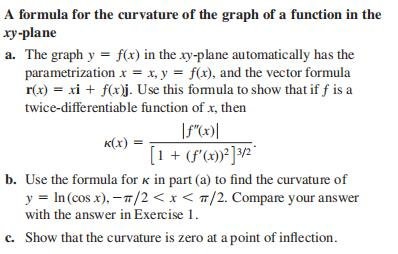### Still have math questions?

Trigonometry
QuestionA formula for the curvature of the graph of a function in the $$x y$$ -plane

a. The graph $$y = f ( x )$$ in the $$x y$$ -plane automatically has the parametrization $$x = x , y = f ( x )$$ , and the vector formula $$r ( x ) = x i + f ( x ) j$$ . Use this formula to show that if $$f$$ is a twice-differentiable function of $$x$$ , then $$\kappa ( x ) = \frac { | f ^ { \prime \prime } ( x ) | } { [ 1 + ( f ^ { \prime } ( x ) ) ^ { 2 } ] ^ { 3 / 2 } }$$ .

$$\text { (b) } \cos x$$